# Question:Is there a more versatile way to draw the curve on the graph?

## Question:Is there a more versatile way to draw the curve on the graph?

Maple 2020

I read the code in the link below and have some thoughts on drawing the graph.

https://www.mapleprimes.com/questions/216092-Options-For-Graph-Drawing-In-The-GraphTheory

restart:
with(GraphTheory):
with(SpecialGraphs):
G     := PetersenGraph();:
POS := GetVertexPositions(G);
EG   := Edges(G);
PLOT(
seq( CURVES([POS[EG[i]], POS[EG[i]]], LINESTYLE(3) ), i=1..numelems(Edges(G))),
POINTS(POS, SYMBOL(_SOLIDBOX, 35), COLOR(RGB, 1, 1, 0)),
seq(TEXT(POS[i], i), i=1..numelems(Vertices(G))),
AXESSTYLE(NONE)
)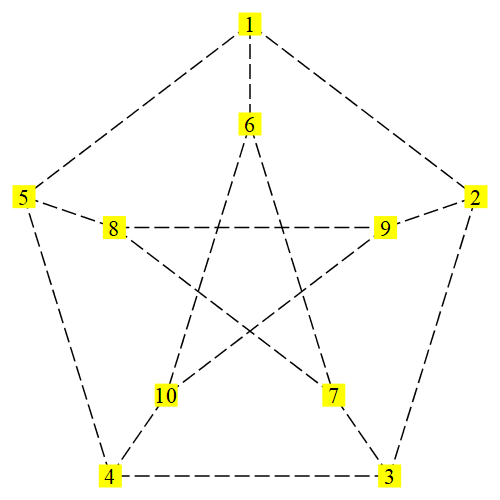But if we want to change the edge to a curvilinear style, it seems very difficult. We know  PetersenGraph is 1-planar graph.  The following picture is one of  its 1-plane drawing.  That is  also what I want to draw .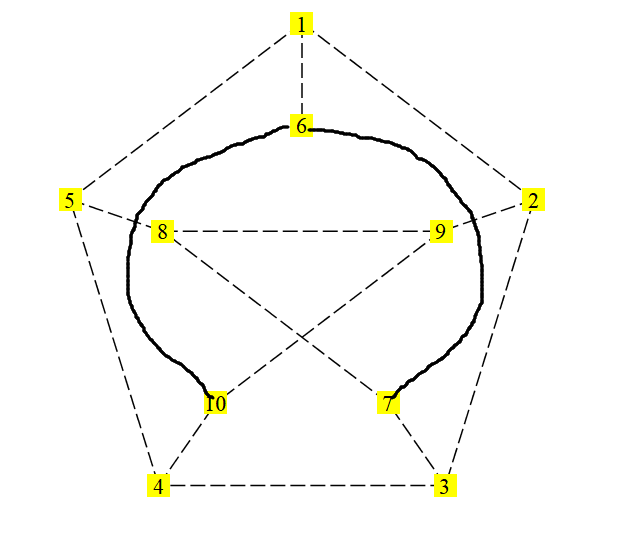PS: 1-planar graph is a graph that can be drawn in the Euclidean plane in such a way that each edge has at most one crossing point, where it crosses a single additional edge

Although I asked a similar question, but it uses too many special curve functions and the method is relatively isolated. Because the cycle graph is too special.  https://www.mapleprimes.com/questions/228987--Can-We-Draw-Curved-Edges-In-The-Graph

I also noticed the optional items of the edge style.  But there are no curves, such as arcs, parabola, etc.

The plot style must be one of line, point, pointline, polygon (patchnogrid), or polygonoutline (patch).

I am trying to draw a parabola, it seems to be quite difficult. And it completely deviated from the use of graph theory package.

drawarc :=proc(A,B,C,ecolor);

local  c,ax,cx ,ay,cy,ox,oy,oo,x,y,b,a,an_end,an_start,r,yuanhu:
ax :=evalf(geometry)[HorizontalCoord](A):
cx :=evalf(geometry)[HorizontalCoord](C):
ay :=evalf(geometry)[VerticalCoord](A):
cy :=evalf(geometry)[VerticalCoord](C):

geometry[circle](c,[ A ,B ,C], [x,y],'centername' =o):

ox :=evalf(geometry[HorizontalCoord] (o)):
oy :=evalf(geometry[ VerticalCoord] (o)):

if(cx -ox)>0 then
b :=arctan((cy -oy) /(cx -ox)):
elif(cx -ox)=0 and (cy -oy)>0 then

b :=Pi/2 :
elif(cx -ox)=0 and (cy -oy)<0 then

b :=-Pi/ 2 :
else
b :=Pi +arctan((cy -oy) /(cx -ox)):
fi :
if(ax -ox)>0 then
a :=arctan((ay -oy) /(ax -ox)):
elif(ax -ox)=0 and (ay -oy)>0 then

a :=Pi /2 :
elif(ax -ox)=0 and (ay -oy)<0 then

a :=-Pi/ 2 ;
else
a :=Pi +arctan((ay -oy)/ (ax -ox)):
fi ;
if(evalf(b)<evalf(a))then
an_start :=a :
else
an_start :=a +2*Pi :
fi :
an_end :=b :

yuanhu :=plottools[ arc] ([ ox , oy] , r , an_start..an_end):

plots[ display] (yuanhu , scaling =constrained, color =ecolor,axes=none);

end :

geometry[point] (a , -3 .00 , 1 .70);
geometry[point] (b , 0 .35 , -0 .45);
geometry[point] (c , 3 .13 , 1 .86);
l :=[a , b , c] ;
drawarc(a,b,c,blue);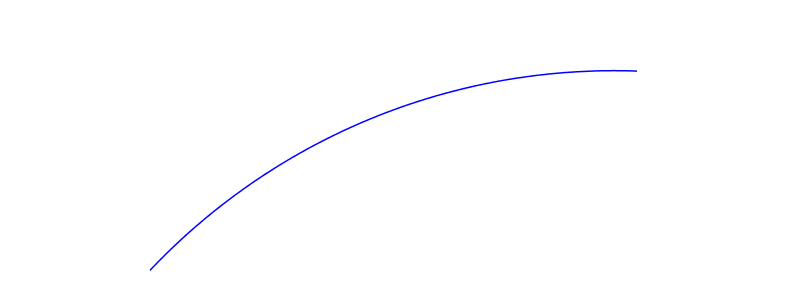I would like to ask maple if there is a more versatile and simpler way to draw a curve of  graph drawing.

drawarcs:=proc(VL ::list , ecolor , scope);
local i , num , arcs , arc_text , vl , display_set ;
vl :=VL ;
num:=nops(vl);
arcs :={};
i:=1;
if num <3 then
"There isn' t enough points in the point list." ;
return ;
elif irem(num-1 , 2)<>0 then
"The number of the list must be multiple of 2 when it minus 1 .";
fi :
while i <num do
arcs :={drawarc(vl[i] ,vl[i +1] , vl[i +2],ecolor),op(arcs)};
i:=i +2 ;
od ;
arc_text :=geometry[ draw] ({vl , vl[num] }, printtext =true , color =ecolor);
display_set:={op(arcs),arc_text};
plots[ display] (display_set , view =[ -abs(scope)..abs(scope), -abs(scope)..abs(scope)] , scaling =constrained,axes=none);
end proc:
geometry[ point] (v1 , 0 ,0):
geometry[ point] (a , 3, 9):
geometry[ point] (b , 7, 9):
geometry[ point] (c, 6,8):
geometry[ point] (d , 12,9):
geometry[ point] (e , 7 ,2):
geometry[ point] (v3 , 9 ,0):
vl:=[ v1,a,b,c,d,e,v3] :
drawarcs(vl,red,20);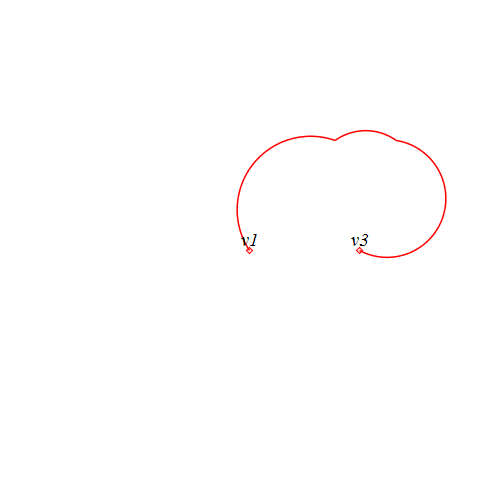try.mwtry.mw

The above program is too cumbersome and not robust

macro(GS=GetVertexPositions):
with(GraphTheory):
with(SpecialGraphs):
G     := PetersenGraph():
POS := GetVertexPositions(G):
EG   := Edges(G) minus {{6,10},{6,7}}:
s1:=PLOT(
seq( CURVES([POS[EG[i]], POS[EG[i]]], LINESTYLE(3) ), i=1..numelems(Edges(G))-2),
POINTS(POS, SYMBOL(_SOLIDBOX, 35), COLOR(RGB, 1, 1, 0)),
seq(TEXT(POS[i], i), i=1..numelems(Vertices(G))),
AXESSTYLE(NONE)
):
geometry[ point] (a,op(GS(G))):
geometry[ point] (b , op(GS(G))):
geometry[ point] (c , op(GS(G))):
geometry[ point] (d ,op(GS(G))+0.3,op(GS(G))-0.5):
geometry[ point] (d2 ,op(GS(G))-0.3,op(GS(G))-0.5):
s2:=drawarc(a,d,b,red):
s3:=drawarc(c,d2,a,red):
plots:-display({s1,s2,s3});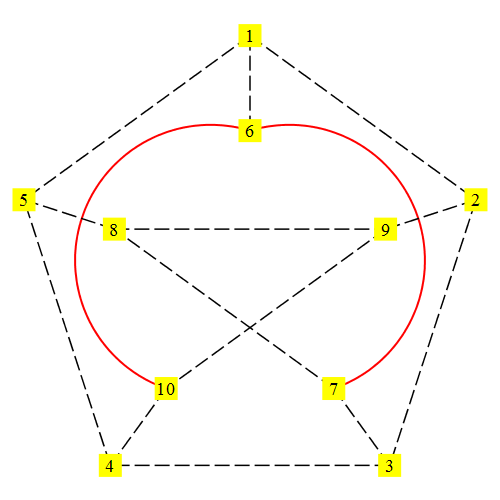﻿